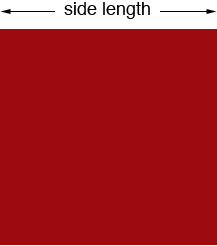SEARCH HOMEMath Central Quandaries & QueriesQuestion from laura: if i had a square of 2.3 square meters what would its radius be?(in centimeters please)Hi Laura,

The area of a square is (the side length) × (the side length).If the square has an area of 2.3 square meters then

(the side length) × (the side length) = 2.3 square meters.

or

(the side length)2 = 2.3 square meters.

To find the side length I used the square root button on my calculator (√) and found that √2.3 = 1.51678 meters.

There are 100 centimeters in a meter so the side length is 151.68 centimeters.

PennyMath Central is supported by the University of Regina and The Pacific Institute for the Mathematical Sciences.